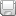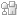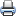# WDM Network DesignSaveDiggDel.icio.usPrint

### Chapter Description

This sample chapter explores some of the design constraints involved in the WDM network design.

## Factors That Affect System Design

Initially, fiber loss was considered the biggest factor in limiting the length of an optical channel. However, as data rates grew and pulses occupied lesser and lesser time slots, group velocity dispersion and nonlinearities (SPM, XPM, and FWM) became important considerations.

As we will see in the following sections, an optical link is designed by taking into account a figure of merit, which is generally the bit error rate (BER) of the system. For most practical WDM networks, this requirement of BER is 10-12 (~ 10-9 to 10-12), which means that a maximum one out of every 1012 bits can be corrupted during transmission. Therefore, BER is considered an important figure of merit for WDM networks; all designs are based to adhere to that quality.

In Chapter 2, we saw the analytical explanation behind BER. It showed BER to be a ratio of the difference of high and low bit levels (power) to the difference in standard deviation of high and low bit levels. As can be observed it is quite difficult to calculate BER instantaneously.

Another plausible explanation of BER can be considered as follows. For a photodetector to detect a 1 bit correctly (assuming nonreturn-to-zero/return-to-zero, or NRZ/RZ modulation; see Chapter 2), it needs a certain minimum number of photons (Np) falling on it. If NTP is the number of photons launched at the transmitter and Δp is the number of photons lost (hypothetically) due to attenuation, absorption, scattering, and other impairments during transmission, then if NTP - Δp < Np, the receiver will not be able to decode the signals properly. To sustain good communication, it is imperative that NTP – Δp > Np over 'L' the desired length of transmission channel. The number of photons translates to the power (which is a function of intensity) of the optical signal.

From the explanation, it becomes evident why optical system design considers power budget and power margins (safety margins for good design) so important.

As far as the dispersion issue goes, we know that dispersion is the spreading of a pulse in time domain, generally due to the large variance of the spectral domain. (Many different spectral components exist in a pulse, each travelling at a different speed.) That means dispersion causes pulse spreading.

The most harmful effect of this pulse spreading is ISI. Even if you assume ISI never to happen (due to good design), still a small amount of dispersion has several harmful effects. The spreading of a pulse lowers its power content, which means that Δp increases. In other words, the number of photons that will strike the photodetector decreases. Therefore, when we are considering dispersion-limited systems, we must consider a power penalty due to dispersion. This power penalty3 can qualitatively be defined as the net loss in power because of dispersion during transmission of a signal in a dispersion affected/limited system.

Qualitatively, power penalty can also be considered as the net extra power required to pump up the signal so that it reaches the receiver (photodetector) while maintaining the minimum BER requirement of the system. Typically, the power penalty for most networks is in the range of 2–3 dB. ITU specification G957 states that this penalty should not be greater than 2 dB.

### Long-Haul Impairments: Nonlinearity

By placing optical amplifiers, we can greatly enhance the power of an optical signal as it reaches the photodetector. Yet another system design consideration is the net fiber nonlinearity that is present in silica fibers. The intensity of the electromagnetic wave propagating through a fiber gives rise to nonlinearities. The refractive index has a strong nonlinear component that depends on the power level of the signal. Nonlinearity produces a nonlinear phase shift denoted by φNL. This is shown in Equation 4-3.

#### Equation 4-3In Equation 4-3,is the nonlinear coefficient that is denoted by Equation 4-4.

#### Equation 4-4In Equation 4-4, n2 is the cladding index, and Aeff is the area of cross-section of the core. Further φNL being dependent of Pin such that Pin by itself is a time varying response. Therefore, the nonlinear phase shift induced in a fast-moving optical pulse is quite dynamic.

The implication is that a frequency chirp is associated with this phase shift. In other words, a pulse at frequency ω0 would, in time, have components in the frequency range shown in the next equation.

ω0 ± φNL

In the equation, φNL is dynamic. The result is pulse spreading, which is a result of the power dependence on the induced phase shift. Therefore, to keep a check on the maximum phase shift that a pulse can have, it is imperative to set a threshold to the maximum input power. This nonlinear phase shift is Self Phase Modulation (SPM). In optical communication, lightpaths need to be designed, keeping in mind the maximum tolerable phase shift φNL < 1. Therefore, the maximum power [Pin max | φNL < 1] can limit phase shift to less than the system requirement.

SPM does not act alone. In optical communication, GVD and SPM often go hand in hand, acting quasi-simultaneously over a length of the fiber. The input channel power needs to be optimized so that it ensures a net dispersion (at a given bit rate) that is less than the minimum tolerable dispersion, as well as for which the net nonlinear effects are under control. In other words, a tradeoff is involved: We need some more power to take care of the dispersion-induced power penalty, but this additional power leads to fiber nonlinear effects (such as SPM), which creates more spread.

An optimization technique involves simulation, whereby we can correctly design the network by considering all the affecting factors and using the appropriate entities to com-pensate for these factors. In one method, SPM and GVD are both calculated on a split Fourier transform. Using this method, frequency domain analysis of the two effects is accomplished by breaking the cylindrical waveguide (fiber) into infinitesimal overlapping segments, such that SPM is assumed to act on odd segments and GVD is set to act on even segments. The final sum of effects on the last and penultimate segments gives the net impairment in the system.

So far, nonlinearities have been considered on just one channel. What happens when we have a WDM system? Do parallel channels have an effect on each other? Two or more channels have nonlinear effects on each other: XPM and FWM. Cross phase modulation results from the different carrier frequency of independent channels, including the associated phase shifts on one another. Cross phase modulation is severely harmful and is twice as powerful as Self phase modulation. The induced phase shift is due to the "walkover" effect, whereby two pulses at different bit rates or with different group velocities walk across each other. The slower pulse sees the walkover and induces a phase shift because of this walkover effect. The total phase shift depends on the net power of all the channels and on the bit output of the channels. Maximum phase shift is produced when two 1 bits walk across each other due to the high power in both the bits (as opposed to the lower power levels when both bits are not at logical 1).

Mathematically, the phase shift is shown in Equation 4-5.

#### Equation 4-5In Equation 4-5, w is the total number of channels and Pk is the power of the kth channel. The maximum phase shift (for all 1 bits) is shown in Equation 4-6.

#### Equation 4-6NOTE

Another method is to solve Schröinger's nonlinear propagation equation shown in the next equation.3. Effect of Chromatic Dispersion on Transmission Length and Induced Power Penalty | Next Section Previous Section